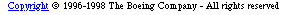+ Text Only Site
+ Non-Flash Version
+ Contact GlennForce for Takeoff Problem or "Will the airplane get off the ground?"
If so instructed by your teacher, print out a worksheet page for these problems.

Materials needed:

• Graphing calculator such as TI-83 or TI-92.

The situation:

Given a Boeing 737 carrying 150 passengers:

Use a weight of 140000 pounds for the Boeing 737. This weight allows for the inclusion of the internal components of the airplane (engine, fuel tanks, landing gear, etc.), the total weight of the passengers, and the total weight of their luggage and cargo.Access FoilSim and set the following conditions:

 Airspeed: 50 mph Altitude: 0 Angle: 5 Thickness: 12.5% Camber: 5.0% Area: 1000 sq ft.

Creating the data set:

Input various values for the airspeed of the airplane. Each time an airspeed is entered, FoilSim will calculate the lift. Generate a set of airspeed and lift values. Record these values on the table on the Forces for Takeoff Worksheet or make a printout of them.

When the set of values is complete, enter these values into the calculator using the stats or data mode.

Using the data set:

Step 1.

Create a scatter plot comparing airspeed and lift. Consider the look of the plot and determine a regression equation that seems to best fit. State an opinion as to:

1. the "meaning" of the graph;
2. the appropriateness of the fit;
3. the variation relationship between airspeed and one of the components that determines lift;
4. the appropriateness and the ease of predicting when the airplane will be able to take off based on the plot and graph.

Step 2.

The amount of force it will take to lift the Boeing 737 off the ground can be calculated using Net Force = Lift - Weight. Create the third column of the calculator "spreadsheet" by calculating the respective net force values. There is an easy way to do this. State (in writing) the method used to create this set of values.

Step 3.

Turn off the "airspeed vs. lift" plot and graph. Then create a scatter plot comparing airspeed and net force. Consider the "airspeed vs. net force" plot. State an opinion on:

1. The "meaning" of the plot.
2. The comparison or contrast between the plot and the "airspeed vs. lift" plot.
3. The appropriateness and the ease of predicting when the airplane will be able to take off based on the plot.
4. The appropriateness or need for creating a "best fit" equation and graph for the "airspeed vs. net force" plot.

Step 4.

Turn off the "airspeed vs. net force" plot (and graph if created). Turn on the "airspeed vs. lift" graph. Then enter an equation for the total weight of the airplane and its passengers. Find the intersection of these two graphs. What is the meaning of the intersection?

Step 5.

Keep the graph of "airspeed vs. lift" and the graph of the total weight turned on. Turn on the plot or graph of the "airspeed vs. net force." Compare/contrast these graphs.

Step 6.

Summarize the information provided by the "airspeed vs. lift" and the "airspeed vs. net force" plots or graphs. Include the following:

1. Which plot is more easily used to picture when the airplane will leave the ground?
2. Which plot provides a better understanding of the other plot?
3. Give the information provided by the equation that may not be provided by the scatter plot.
4. Which type of variation does each situation model?
5. What can you observe about the coefficients of the equations?
6. Print out copies of tables, plots, and graphs to support your summary.

Step 7.

Use the World Wide Web to access the Forces on an Airplane slide. Compare and contrast the lift and the net force needed to allow an aircraft to leave the ground. Describe the factors that affect lift.

Related Pages:
Standards
Worksheet
Lesson Index
Aerodynamics Index+ Inspector General Hotline + Equal Employment Opportunity Data Posted Pursuant to the No Fear Act + Budgets, Strategic Plans and Accountability Reports + Freedom of Information Act + The President's Management Agenda + NASA Privacy Statement, Disclaimer, and Accessibility CertificationEditor: Tom Benson NASA Official: Tom Benson Last Updated: Thu, May 13 02:38:38 PM EDT 2021 + Contact Glenn